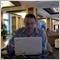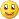# Tick Indicator and EAs - page 5Moderator
119020

Several months ago I wanted to use scalping with asctrend system in elite section. I described Igorad the following:

- let's imagine that price is the vehicle driving by some crazy driver without speedometer inside the vehicle. So, the driver does not know the speed. Vehicle is stopping trying to change the direction, or moving too fast sometimes and coming back and so on. Is it possible to create Speedometer indicator for this vehicle?So he created this indicator (attached). You may see everything with new ticks. I tried it but realized later that it should be a system with many indicators and one indicator is not enough.

Later on Kalenzo or Raff coded some good indicator to estimate the speed (in public thread somewhere) but not for scalping sorry.

Later on somebody created MACD_ticks (not exact name of the indicator, sorry) and so on. It was posted on some public thread as well.

Files:1310

Modified Speedometer

Files:
speed18.gif 29 kb127

newdigital:
Several months ago I wanted to use scalping with asctrend system in elite section. I described Igorad the following:

- let's imagine that price is the vehicle driving by some crazy driver without speedometer inside the vehicle. So, the driver does not know the speed. Vehicle is stopping trying to change the direction, or moving too fast sometimes and coming back and so on. Is it possible to create Speedometer indicator for this vehicle?Could you explain how you would have considered using it as a system for scalping. The use I make of it is only as a filter, meaning, not to take the trade when to slow, or exit when the move against your profit is too fast.Moderator
119020

dav12028:
Could you explain how you would have considered using it as a system for scalping. The use I make of it is only as a filter, meaning, not to take the trade when to slow, or exit when the move against your profit is too fast.

I tried to use it and realized that we need some more indicators same as ma_speedometer, MACD_speedometer and so on. It should be trading system. One indicator is not enough.1310

Files:1310

Files:1310

Kalman Filtering

p-position

v-velocity

a-random, time-varying acceleration

T-is the time between step k and step k+1

The question which is addressed by the Kalman filter is this: Given our knowledge of the behavior of the system, and given our measurements, what is the best estimate of position and velocity?

MATLAB source:

==============================

function kalman(alpha, duration, dt)

% function kalman(alpha, duration, dt) - Kalman filter simulation

% alpha = forgetting factor (alpha >= 1)

% duration = length of simulation (seconds)

% dt = step size (seconds)

measnoise = 10; % position measurement noise (feet)

accelnoise = 0.5; % acceleration noise (feet/sec^2)

a = [1 dt; 0 1]; % transition matrix

c = [1 0]; % measurement matrix

x = [0; 0]; % initial state vector

xhat = x; % initial state estimate

Q = accelnoise^2 * [dt^4/4 dt^3/2; dt^3/2 dt^2]; % process noise covariance

P = Q; % initial estimation covariance

R = measnoise^2; % measurement error covariance

% set up the size of the innovations vector

Inn = zeros(size(R));

pos = []; % true position array

poshat = []; % estimated position array

posmeas = []; % measured position array

Counter = 0;

for t = 0 : dt: duration,

Counter = Counter + 1;

% Simulate the process

ProcessNoise = accelnoise * [(dt^2/2)*randn; dt*randn];

x = a * x + ProcessNoise;

% Simulate the measurement

MeasNoise = measnoise * randn;

z = c * x + MeasNoise;

% Innovation

Inn = z - c * xhat;

% Covariance of Innovation

s = c * P * c' + R;

% Gain matrix

K = a * P * c' * inv(s);

% State estimate

xhat = a * xhat + K * Inn;

% Covariance of prediction error

P = a * P * a' + Q - a * P * c' * inv(s) * c * P * a';

% Save some parameters in vectors for plotting later

pos = [pos; x(1)];

posmeas = [posmeas; z];

poshat = [poshat; xhat(1)];

end

% Plot the results

t = 0 : dt : duration;

t = t';

plot(t,pos,'r',t,poshat,'g',t,posmeas,'b');

grid;

xlabel('Time (sec)');

ylabel('Position (feet)');

title('Kalman Filter Performance');

Files:
kalman5.gif 2 kb
kalman.pdf 426 kb1310

Kalman Filtering indicator

Index of /Forex/BlackBoxes/Kalman

Files:
kalman.zip 25 kb
kalman1.gif 26 kb1310

Kalman filter toolbox for Matlab

Example of Kalman filtering a particle moving in the plane at constant velocity

~~~~~~~~~~~~~~~~~~~~~~~~~~~~~~~~~~~~~~~~~~~~~~~

Here is a simple example. Consider a particle moving in the plane at constant velocity subject to random perturbations in its trajectory. The new position (x1, x2) is the old position plus the velocity (dx1, dx2) plus noise w.

[ x1(t) ] = [1 0 1 0] [ x1(t-1) ] + [ wx1 ]

[ x2(t) ] [0 1 0 1] [ x2(t-1) ] [ wx2 ]

[ dx1(t) ] [0 0 1 0] [ dx1(t-1) ] [ wdx1 ]

[ dx2(t) ] [0 0 0 1] [ dx2(t-1) ] [ wdx2 ]

We assume we only observe the position of the particle.

[ y1(t) ] = [1 0 0 0] [ x1(t) ] + [ vx1 ]

[ y2(t) ] [0 1 0 0] [ x2(t) ] [ vx2 ]

[ dx1(t) ]

[ dx2(t) ]

~~~~~~~~~~~~~~~~~~~~~~~~~~~~~~~~~~~~~~~~~~~208

hi

hi

very intresting thing. can you post an example chart how you trade this. I read your explanation but not easy to get it. would be nice to sse some examples.

thx

lodol